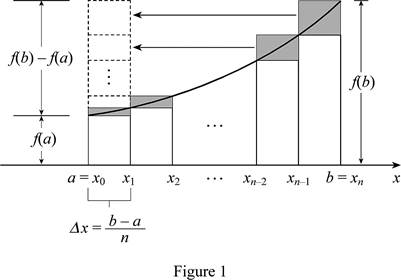# The relation between area, lower sum, and upper sum for an increasing function f .### Single Variable Calculus: Concepts...

4th Edition
James Stewart
Publisher: Cengage Learning
ISBN: 9781337687805### Single Variable Calculus: Concepts...

4th Edition
James Stewart
Publisher: Cengage Learning
ISBN: 9781337687805

#### Solutions

Chapter 5.1, Problem 23E

(a)

To determine

## To find: The relation between area, lower sum, and upper sum for an increasing function f.

Expert Solution

The relation between area, lower sum, and upper sum for a function is Ln<A<Rn_ .

### Explanation of Solution

Given information:

The curve f is an increasing continuous function.

Describe the relation between Ln , A, and Rn as below:

• The increasing function f represents the lower sum (Ln) and an upper sum (Rn) . So Ln is an underestimate of area (A) and Rn is an overestimate of area (A).
• The value of Rn is more than the area A and the value of Ln is less than the area A.

Therefore, the terms are related as Ln<A<Rn_ .

(b)

To determine

### To prove: The relation Rn−Ln=b−an[f(b)−f(a)] .

Expert Solution

The relation RnLn=ban[f(b)f(a)] is proved.

### Explanation of Solution

The expression for upper estimate of area is shown below:

Rn=f(x1)Δx+f(x2)Δx+...+f(xn)Δx (1)

Here, the right endpoint height of the first rectangle is f(x1) , the width of the interval is Δx , the right endpoint height of the second rectangle is f(x2) , and the right endpoint height of nth rectangle is f(xn) .

The expression for lower estimate of area is shown below:

Ln=f(x0)Δx+f(x1)Δx+...+f(xn1)Δx (2)

Here, the left endpoint height of the first rectangle is f(x0) , the left endpoint height of the second rectangle is f(x1) , and the left endpoint height of nth rectangle is f(xn1) .

Subtract Equation (2) from Equation (1) as shown below:

RnLn=[[f(x1)Δx+f(x2)Δx+...+f(xn)Δx][f(x0)Δx+f(x1)Δx+...+f(xn1)Δx]]=f(xn)Δxf(x0)Δx=Δx[f(xn)f(x0)] (3)

The width of the interval (Δx) is expressed as shown below:

Δx=ban (4)

Here, the upper limit is b, the lower limit is a, and the number of rectangles is n.

Substitute b for xn , a for x0 , and ban for Δx in Equation (3).

RnLn=ban[f(b)f(a)]

Hence, the relation is proved.

Draw the diagram for an increasing function f with n rectangles as shown in Figure (1).Refer to Figure (1).

• The difference in upper sum and lower sum (RnLn) represents the sum of areas of the shaded rectangles.
• Slide the shaded rectangles to the left and stack it on the top of the leftmost shaded rectangle.
• The stacked rectangles forms a rectangle with height f(b)f(a) and width Δx=ban .
• Hence, the area is ban[f(b)f(a)] .

Therefore, the estimate RnLn is reassembled and forms a single rectangle with area of ban[f(b)f(a)] .

(c)

To determine

### To prove: (Rn−A) is less than b−an[f(b)−f(a)] .

Expert Solution

Yes, the expression is proved.

### Explanation of Solution

Refer to part (a).

The value of Ln is less than the area A.

Therefore, it can be expressed as A>Ln . Also it is valid for the inequality RnA<RnLn .

Substitute ban[f(b)f(a)] for RnLn .

RnA<ban[f(b)f(a)]

Therefore, the expression is valid and it is proved.

### Have a homework question?

Subscribe to bartleby learn! Ask subject matter experts 30 homework questions each month. Plus, you’ll have access to millions of step-by-step textbook answers!